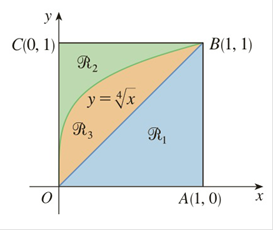Chapter 5.2, Problem 19E

Chapter
Section
Textbook Problem

# Refer to the figure and find the volume generated by rotating the given region about the specified line.ℛ 1 about OA

To determine

To find:

The volume generated by rotating the region R1 about theline  OA by using the figure.

Explanation

1)  Concept:

i. If the cross section is a disc and the radius of the disc is in terms of x  or y, then the area

ii. The volume of the solid revolution about the x-axis is

V= abA(x)dx

2) Given:

Figure:

3) Calculation:

Here, the line OB appears to be the line y=x and line OA appears to be the line  y=0.

On rotating R1 about OA that is the line  y=0, the cross section is perpendicular to the x-axis. And it is a disc with radius r=x because it is the distance from the line OB(y=x)  to the axis of rotationOA (y=0).

From the figure, the solid lies between x=0 and  x=1

### Still sussing out bartleby?

Check out a sample textbook solution.

See a sample solution

#### The Solution to Your Study Problems

Bartleby provides explanations to thousands of textbook problems written by our experts, many with advanced degrees!

Get Started

#### Find more solutions based on key concepts